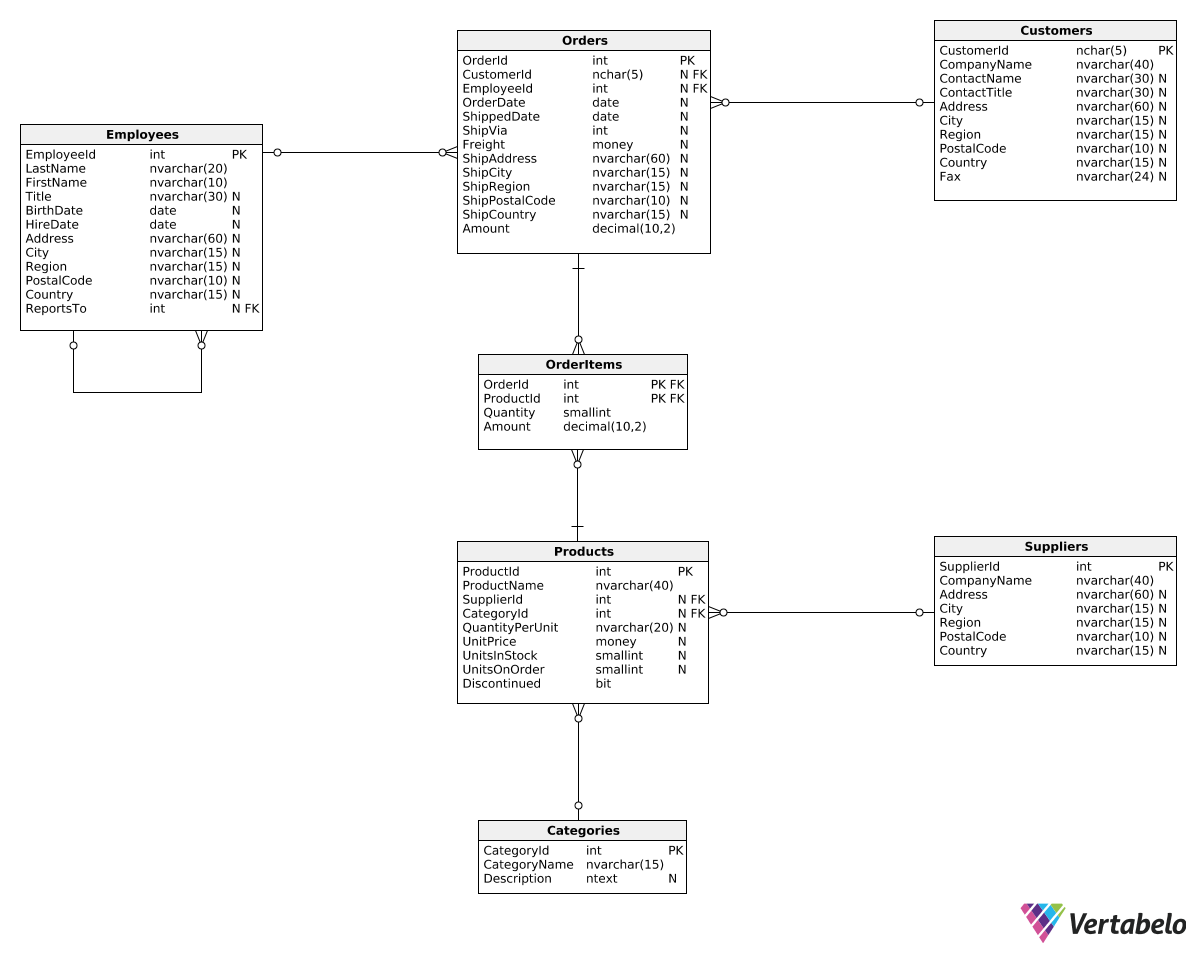Deals Of The Week - hours only!Up to 80% off on all courses and bundles.-Close
Introduction
Total revenue for multiple periods
Calculating deltas
Creating "revenue in quarters" reports
Summary

## Instruction

Good job! Now that we know how to calculate the revenue value for the previous period, we can easily calculate the revenue change (delta) between two periods. Take a look:

SELECT
DATEPART(Year, OrderDate) AS RevenueYear,
SUM(Amount) AS TotalRevenue,
SUM(Amount) - LAG(SUM(Amount), 1) OVER (ORDER BY DATEPART(Year, OrderDate)) AS Delta
FROM Orders
GROUP BY DATEPART(Year, OrderDate)
ORDER BY DATEPART(Year, OrderDate);


Result:

RevenueYear TotalRevenue Delta
2016 208083.98 null
2017 617085.21 409001.23
2018 440623.90 -176461.31

In the Delta column, we simply subtracted the revenue generated in the previous year from the revenue generated in the current year. Once again, we used the LAG() function pattern from the previous exercise.

## Exercise

Show the total revenue for each quarter in each year alongside the delta (revenue change) as compared to the previous quarter. Show the following columns: RevenueYear, RevenueQuarter, TotalRevenue, and Delta.

In the first row, leave the delta value as NULL. Order the rows by year and quarter.

### Stuck? Here's a hint!

Modify the query from the explanation. Use DATEPART(Year, OrderDate) and DATEPART(Quarter, OrderDate) in the SELECT, OVER(), and GROUP BY clauses.